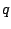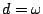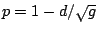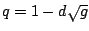Next: Band-pass filter Up: Designing filters Previous: One-pole, one-zero high-pass filter   Contents   Index

## Shelving filter

Generalizing the one-zero, one-pole filter above, suppose we place the zero at a point, a real number close to, but less than, one. The pole, at the point, is similarly situated, and might be either greater than or less than, i.e., to the right or left, respectively, but with bothandwithin the unit circle. This situation is diagrammed in Figure 8.14.At points of the circle far fromand, the effects of the pole and the zero are nearly inverse (the distances to them are nearly equal), so the filter passes those frequencies nearly unaltered. In the neighborhood ofand, on the other hand, the filter will have a gain greater or less than one depending on which oforis closer to the circle. This configuration therefore acts as a low-frequency shelving filter. (To make a high-frequency shelving filter we do the same thing, only placingandclose to -1 instead of 1.)

To find the parameters of a shelving filter given a desired transition frequency(in angular units) and low-frequency gain, first we choose an average distance, as pictured in the figure, from the pole and the zero to the edge of the circle. For small values of, the region of influence is aboutradians, so simply setto get the desired transition frequency.

Then put the pole atand the zero at. The gain at zero frequency is thenas desired. For example, in the figure,is 0.25 radians andis 2.Next: Band-pass filter Up: Designing filters Previous: One-pole, one-zero high-pass filter   Contents   Index
Miller Puckette 2006-12-30